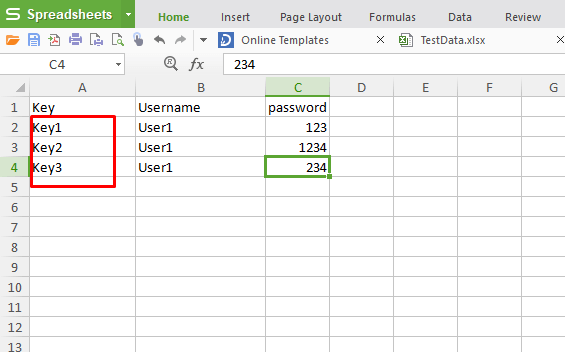# How to Read data from excel in selenium C#

During the automation some time we need to read data from excel using selenium c#. There are multiple way to read data from excel in selenium c#. Let’s see one by one. In the Example 1 will see how to read data from excel by using key. In example 2 will see how to read data from a cell by using the column and row matrix.

Read data from excel in selenium using c# by key.

Example 1: Suppose we have a excel file with name “TestData.xlxs”.

Create a connection with excel file in selenium C#.

```publicstaticstring TestDataFileConnection()
{
var path = System.IO.Path.GetDirectoryName(System.Reflection.Assembly.GetExecutingAssembly().GetName().CodeBase);
path = path.Substring(6) + @"\Data\TestData.xlsx";
var con = string.Format(@"Provider=Microsoft.ACE.OLEDB.12.0;Data Source = {0}; Extended Properties=Excel 12.0;", path);
return con;
}
```Read data from excel in selenium C#

```publicstatic UserData GetTestData(string keyName)
{
using (var connection = new OleDbConnection(TestDataFileConnection()))
{
connection.Open();
var query = string.Format("select * from [DataSet\$] where key='{0}'", keyName);
var value = connection.Query<UserData>(query).FirstOrDefault();
connection.Close();
return value;
}
}
```

Here the “DataSet” is a sheetname of your excel.

Method calling and output

```var userData = ExcelDataAccess.GetTestData("Admin");
```

Output: user1

Example 2

Read Data from excel in selenium c# using from a cell

```public static String getData(int row, int col)
{
String value;

excel.Application x1app = new excel.Application();

excel.Workbook x1Wb = x1app.Workbooks.Open(@ConfigurationManager.AppSettings["TestDataPath"]);
//excel.Workbook x1Wb = x1app.Workbooks.Open(@"D:\TestDataFile.xlsx");

excel.Worksheet x1Ws = x1Wb.Sheets;

excel.Range x1range = x1Ws.UsedRange;

// value = x1range.Cells[row][col].value2;

value = Convert.ToString(x1range.Cells[row][col].value2);
x1app.Quit();
return value;
}
```

Method calling and output

`String s1 = getData(1,1);`

Output: User1